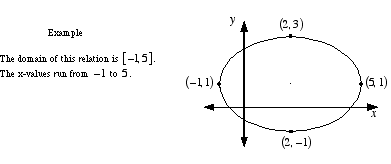index: click on a letter A B C D E F G H I J K L M N O P Q R S T U V W X Y Z A to Z index index: subject areas numbers & symbols sets, logic, proofs geometry algebra trigonometry advanced algebra & pre-calculus calculus advanced topics probability & statistics real world applications multimedia entrieswww.mathwords.com about mathwords website feedback

 Domain The set of values of the independent variable(s) for which a function or relation is defined. Typically, this is the set of x-values that give rise to real y-values. Note: Usually domain means domain of definition, but sometimes domain refers to a restricted domain.See also